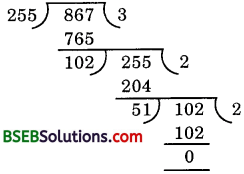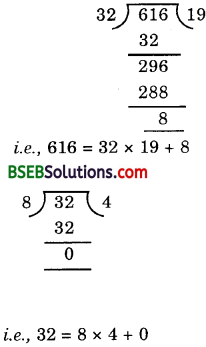# Bihar Board Class 10th Maths Solutions Chapter 1 Real Numbers Ex 1.1

Bihar Board Class 10th Maths Solutions Chapter 1 Real Numbers Ex 1.1 Textbook Questions and Answers.

## BSEB Bihar Board Class 10th Maths Solutions Chapter 1 Real Numbers Ex 1.1

Question 1.
Use Euclid’s division algorithm to find the HCF of:

(i) 135 and 225
(ii) 196 and 38220
(iii) 867 and 255

Solution:

(i) We have:
Dividend = 225 and Divisor = 135
The process can be exhibited as under:Hence, HCF (135, 225) = 45.

(ii) We have:
Dividend = 38220 and Divisor = 196
38220 = 196 × 195 + 0
Hence, HCF (196, 38220) = 196.(iii) We have:
Dividend = 867 and Divisor = 255Hence, HCF (255, 867) = 51.Question 2.
Show that any positive odd integer is of the form 6q + t or 6q + 3 or 6q + 5, where q is some integer.
Solution:
Let a be any positive integer and b = 6. Then, by Euclid’s Division Lemma a = 6q + r, for some integer q ≥ 0, and where 0 ≤ r < 6, the possible remainders are 0, 1, 2, 3, 4 or 5.

That is, a can be 6q or 6q + 1 or 6q + 2 or 6q + 3 or 6q + 4 or 6q + 5, where q is the quotient. If a = 6q or 6q + 2 or 6q + 4, then a is an even integer.

Also, an integer can be either even or odd. Therefore, any odd integer is of the form 6q + 1 or 6q + 3 or 6q + 5, where q is some integer.

Question 3.
An army contingent of 616 members is to march behind an army band of 32 members in parade. The two groups are to march in the same number of columns. What is the maximum number of columns in which they can march?
Solution:
To find the maximum number of columns, we have to find the HCF of 616 and 32.∴ The HCF of 616 and 32 is 8.
Hence, maximum number of columns is 8.Question 4.
Use Euclid’s division lemma to show that the square of any positive integer is either of the form 3m or 3m + 1 for some integer m. [CBSE, Foreign, 2008]
Solution:
Let x be any positive integer. Then it is of the form 3q, 3q + 1 or 3q + 2. Now, we have to prove that the square of each of these can be written in the form 3m or 3m + 1.
Now, (3q)2 = 9q2 = 3(3q2)
= 3m,
where in = 3q2
(3q + 1)2 = 9q2 + 6q + 1
= 3(3q2 + 2q) + 1
= 3m+ 1, where m = 3q2 + 2q
and (3q + 2)2 = 9q2 + 12q + 4
= 3(3q2 + 4q + 1) + 1
= 3m + 1, where m = 3q2 + 4q + 1
Hence, the result.

Question 5.
Use Euclid’s division lemma to show that the cube of any positive integer is either of the form 9q, 9q + 1 or 9q + 8.
Solution:
Let x be any positive integer. Then it is of the form 3m, 3m + 1 or 3m + 2. Now, we have to prove that the cube of each of these can be rewritten in the form
9q, 9q + 1 or 9q + 8.
Now, (3m)3 = 27m3 = 9(3m3)
= 9q, where q = 3m3
(3m + 1)3 = (3m)3 + 3(3m)2. 1 + 3(3m). 12 + 1
= 27m3 + 27m2 + 9m + 1
= 9(3m3 + 6m2 + 4m) + 8
= 9q + 8, where q = 3m3 + 6m2 + 4m.

If you face any issues concerning NCERT Bihar Board Class 10 Maths Solutions Chapter 1 Real Numbers Ex 1.1, you can ask in the comment section below, we will surely help you out.